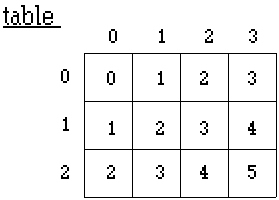Computer Science - C++   Lesson 18 - Two Dimensional Arrays

(apmatrix class)

INTRODUCTION:               Two dimensional arrays allow the programmer to solve problems involving rows and columns.  Many data processing problems involve rows and columns, such as an airplane reservation system or the mathematical modeling of bacteria growth.  A classic problem involving two-dimensional arrays is the bacteria program presented in the lab exercise, Life.  After surveying the syntax and unique aspects of these larger data structures, such information will be applied to more challenging lab exercises.

As with the previous lesson, a standard matrix class will be provided to implement 2-dimensional arrays.  This apmatrix class will support routines similar to those used with the apvector class.

The key topics for this lesson are:

A.  Two-Dimensional Arrays

B.   Passing Two-Dimensional Arrays to Functions

C.  Two-Dimensional Array Algorithms

VOCABULARY:          MATRIX                   ROW            COLUMN

DISCUSSION:                       A.  Two-Dimensional Arrays

1.   The apmatrix class is a two-dimensional version of an array which uses multiple subscripts.  This allows for the creation of table-like data structures with a row and column format.  The first subscript will define a row of a table with the second subscript defining a column of a table.  The apmatrix class will be used to implement 2-D arrays in the curriculum guide.  Here is an example program including a diagram of the array.

Program 18-1

#include <apmatrix.h>

main ()

{

apmatrix<int>  table(3,4);

int  row, col;

for (row = 0; row <3; row++)

for (col = 0; col <4; col++)

table[row][col] = row + col;

return 0;

}2.   The declaration of a apmatrix type is similar to that of an apvector type.  The data type stored in the apmatrix is declared between the <type> placeholders.  The size of the table is specified by the integer arguments inside of the parentheses, i.e. (3,4).

3.   Just as with one-dimensional arrays, the row and column numbering of a 2-D array begin at subscript location zero (0).  The 3 rows of the table are referenced from rows 0..2.  Likewise, the 4 columns of the table are referenced from columns 0..3.

4.   The two-dimensional table is filled with the sums of row and col.  To access each location of the matrix, specify the row coordinate first, then the column:

table [row][col]

Each subscript must have its own square brackets.  Do not use table [row,col] as is used in some other programming languages such as Pascal

B.   Passing Two-Dimensional Arrays to Functions

1.   The following program will illustrate parameter passing of an apmatrix.  The purpose of this program is to read a text file containing integer data, store it in a 2-D array, and print it out.  The contents of the text file "data.txt" is shown first:

"data.txt"                    17  3  2 13

5 10 11  8

9  6  7 12

4 15 14  1

Program 18-2

#include <apmatrix.h>

#include <iomanip.h>

#include <fstream.h>

#include <iostream.h>

// A program to illustrate apmatrix parameter passing

const int MAX = 4;

void printTable (const apmatrix<int> &table);

void loadTable (apmatrix<int> &table);

main ()

{

apmatrix<int> grid(MAX,MAX);

loadTable (grid);

printTable (grid);

return 0;

}

void printTable (const apmatrix<int> &table)

{

for (int row=0; row<MAX; row++)

{

for (int col=0; col<MAX; col++)

cout << setw(4) << table[row][col];

cout << endl;

}

}

void loadTable (apmatrix<int> &table)

{

ifstream inFile("c:\\apcs\\data.txt",ios::in);

for (int row=0; row<MAX; row++)

for (int col=0; col<MAX; col++)

inFile >> table[row][col];

}

2.   The function loadTable uses a reference parameter,

(apmatrix<int> &table).  The local identifier table serves as an alias for the actual parameter grid passed to the function.

3.   The function printTable uses a const reference parameter,

(const apmatrix<int> &table).  This avoids making a local copy of grid, yet guarding the data of grid from any changes.

4.   The apmatrix class performs range-checking on the indexing operator ([]).  If any code attempts to access beyond the boundaries of the matrix, the programs stops.

C.  Two-Dimensional Array Algorithms

1.   The most common 2-D array algorithms will involve processing the entire grid, usually row-by-row or column-by-column.

2.   Problem-solving on a matrix could involve processing:

a.  one row

b.  one column

c.  one cell

d.  adjacent cells in various different directions

3.   In the next lesson we will look at a 2-D recursive solution to a rather difficult problem.

SUMMARY/REVIEW:         Two-dimensional arrays will be applied to two interesting problems.  The simulation of life in a petri dish of bacteria will require a two-dimensional array representation.  The second and third lab exercises are different versions of the "Knight's Tour" problem, an interesting and demanding chess movement problem.

ASSIGNMENT:                   Lab Exercise, L.A.18.1, Life